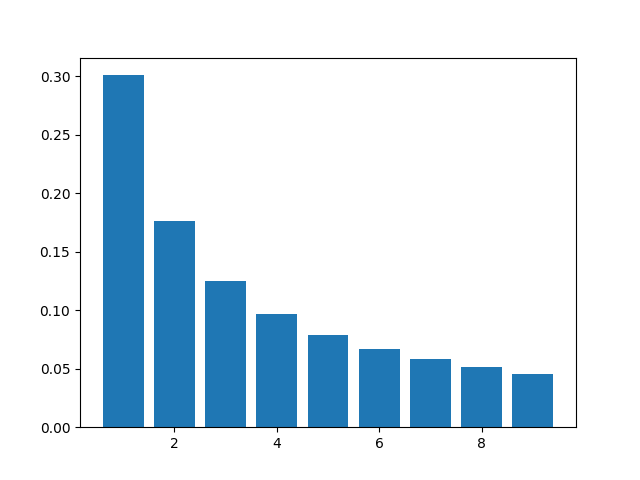How are the first digits of primes distributed? Do some digits appear as first digits of primes more often that others? How should we even frame the problem?

There are an infinite number of primes that begin with each digit, so the cardinalities of the sets of primes beginning with each digit are the same. But cardinality isn’t the right tool for the job.

The customary way to (try to) approach problems like this is to pose a question for integers up to N and then let N got to infinity. This is called natural density. But this limit doesn’t always converge, and I don’t believe it converges in our case.

## Logarithmic density

An alternative is to compute logarithmic density. This measure exists in cases where natural density does not. And when natural density does exist, logarithmic density may give the same result .

The logarithmic density of a sequence A is defined byWe want to know about the relative logarithmic density of primes that start with 1, for example. The relative density of a sequence A relative to a sequence B is defined byWe’d like to know about the logarithmic density of primes that start with 1, 2, 3, …, 9 relative to all primes. The exact value is known , and we will get to that shortly, but first let’s try to anticipate the result by empirical calculation.

We want to look at the first million primes, and we could do that by calling the function prime with the integers 1 through 1,000,000. But it is much more efficient to as SymPy to find the millionth prime and then find all primes up to that prime.

    from sympy import prime, primerange
import numpy as np

first_digit = lambda n: int(str(n))

density = np.zeros(10)
N = 1_000_000
for x in primerange(prime(N+1)):
density += 1/x
density[first_digit(x)] += 1/x
density /= density


Here’s a histogram of the results.Clearly the distribution is uneven, and in general more primes begin with small digits than large digits. But the distribution is somewhat irregular. That’s how things work with primes: they act something like random numbers, except when they don’t. This sort of amphibious nature, between regularity and irregularity, makes primes interesting.

The limits defining the logarithmic relative density do exist, though the fact that even a million terms doesn’t produce a smooth histogram suggests the convergence is not too rapid.

In the limit we get that the density of primes with first digit d isThe densities we’d see in the limit are plotted as follows.This is exactly the density given by Benford’s law. However, this is not exactly Benford’s law because we are using a different density, logarithmic relative density rather than natural density .

## Related posts

 The Davenport–Erdős theorem says that for some kinds of sequences, if the natural density exists, logarithmic density also exists and will give the same result.

 R. E. Whitney. Initial Digits for the Sequence of Primes. The American Mathematical Monthly, Vol. 79, No. 2 (Feb., 1972), pp. 150–152

 R. L. Duncan showed that the leading digits of integers satisfy the logarithmic density version of Benford’s law.